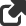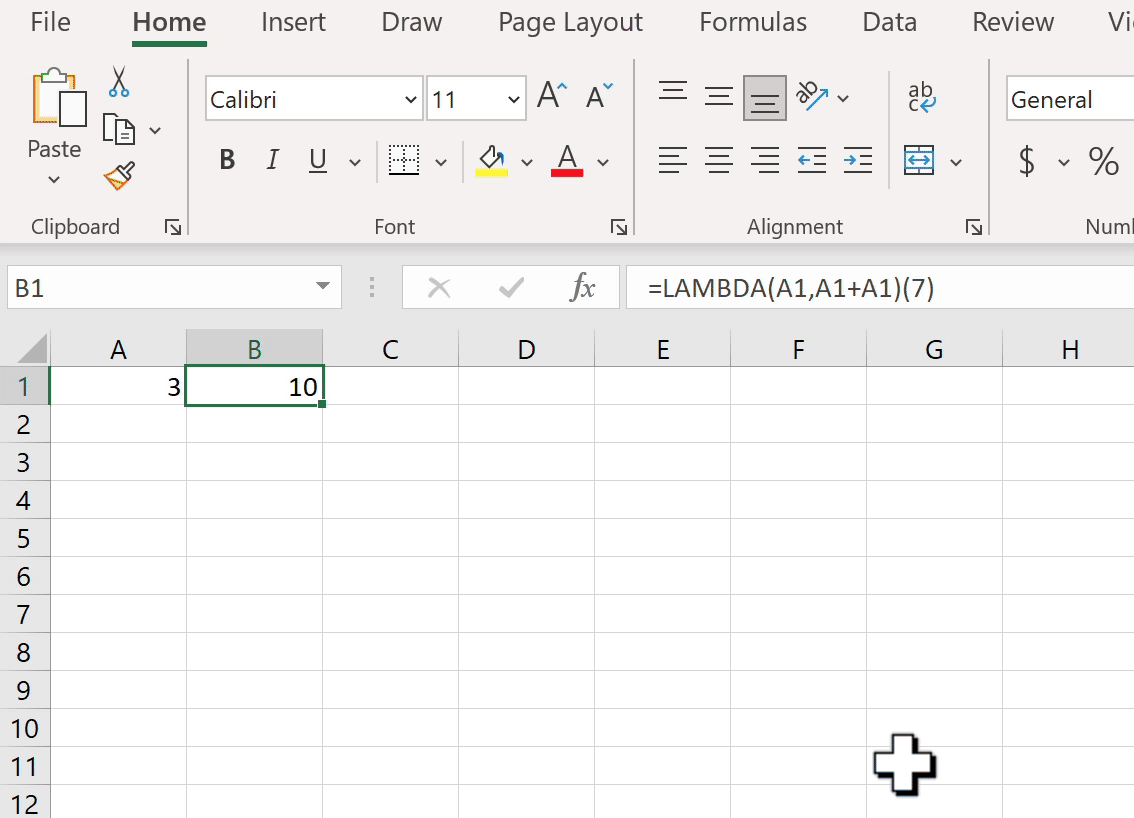# Blog

All Blog Posts  |  Next Post  |  Previous Post

#Lambdas in FlexCel

Bookmarks:

#### Thursday, April 01, 2021As you might know, some months ago Microsoft released support for lambda functions in Excel. It was no small change: Lambdas turned the calculation engine into turing-complete, and they can change your spreadsheets in fundamental ways.

The main reason why you might want lambdas is that they allow you to define user-defined functions in a spreadsheet, using only other formulas. No VBA or macros required. But Lambda functions are much more: they are real first-class functions that you can pass around. They can even do variable captures like a Lambda in C# or an anonymous method in Delphi.

Even with them being only months old, there are a lot of articles and posts about them. So we will not repeat those here, and we will instead focus on a question that we have heard a lot lately: When is FlexCel going to support Lambdas?

And the answer is, of course, today. But wait! Let me look at the date... and let me look at the other post today on this site about running FNC in a Sperry Univac Uniscope 200... What can I say? Lambdas weren't supposed to ship on April 1st; they were supposed to ship weeks ago. But we kept finding small stuff to polish. As it is usual in FlexCel development, we wanted to cover all cases that we could and make sure everything worked as it should. And as it is usual too, we were late and I am writing this post in April 1st.

You can download FlexCel 7.9 on our site, and start adding lambdas and lets everywhere. Just the usual advice: "With great power comes great responsibility". As powerful as they are, it is simple to abuse them and end up with spreadsheets only a mother could love. Or understand. I've already seen pages-long lambdas written in many places, and one has to wonder how you are supposed to debug those formulas once something goes wrong. Right now, Excel tools for debugging lambdas are quite poor.

#### Tales from the trenches

Following a one post tradition, I am reviving the "tales from the trenches" section for this post. This is the section where I write about small details we find in our daily development. Of course, every day has a different tale, so I've chosen one at random to close this entry.

##### Schrödinger parameter names.
When we wrote the validation code for parameter names, we assumed that they would follow the same rules as Excel names. In particular, you can't have a name that is also a valid cell reference. So you can't have a name named "A1" or "R1C1". You can't either name a name "R" or "C" since that means "Full Row" and "Full Column" in R1C1 notation.

But, while you can't name a parameter "A1", you can name it "C". So how does this work? How does Excel know that when I write "=Sum(C)" I refer to the parameter C and not to the entire column?
The only way to find out was to try it, so I set the reference mode in Excel to R1C1, and went to write the formula `=Lambda(C,Sum(C))`

It gave an error of invalid name. So I tried now with `=Lambda(A1,Sum(A1))`, and Excel happily accepted it.

Valid parameter names depend on the reference mode you are using. And we coded FlexCel the same way: If you are in R1C1, FlexCel will allow "a1" as parameter name, but no "C" or "R". If you are in A1 mode, the reverse.

Now, if you are like us, this probably raised even more questions. The formulas are saved to xlsx files as text, but always in A1 reference style.
So imagine we are in Excel in R1C1 mode and write the formula `=LAMBDA(A1, A1 *R1C1)`
As formulas in xlsx files are saved in A1 notation no matter the mode, this would be saved as `=LAMBDA(A1, A1 * A1)`. But the first A1 in the expression is the parameter, and the second is a cell reference. And if you try it, you'll see that Excel figures out which is which.

As you can see below, the formula =Lambda(A1, A1+A1) is evaluating to 10: Cell A1 is 7 and parameter A1 is 3. So the sum is 10.

But if you want to edit the formula, it will not let you, as it won't be able to figure out which A1 is which.Ok, so where is the trick? Because you can't know which one is which one from the formula above. Luckily the answer is simple: the formulas in xlsx are saved as `=xlfn.LAMBDA(xlpm.A1, xlpm.A1 * A1)`, so when reading the file, you can know which ones are parameters and which are cells. And yes, I can hear you say: What if I name a parameter "xlpm.A1"?  The answer is that you can't, because dots are not allowed in parameter names. All bases are covered.

Bookmarks: